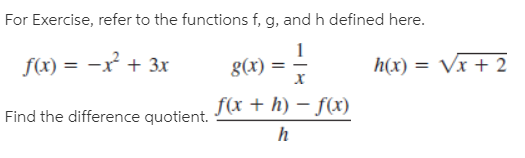# For Exercise, refer to the functions f, g, and h defined here. f(x) = -x + 3x g(x) = h(x) = Vx + 2 %3D Find the difference quotient. f(x + h) – f(x)

Questionhelp_outlineImage TranscriptioncloseFor Exercise, refer to the functions f, g, and h defined here. f(x) = -x + 3x g(x) = h(x) = Vx + 2 %3D Find the difference quotient. f(x + h) – f(x) fullscreen

## Expert Answer

### Want to see this answer and more?

Experts are waiting 24/7 to provide step-by-step solutions in as fast as 30 minutes!*

*Response times vary by subject and question complexity. Median response time is 34 minutes and may be longer for new subjects.
Tagged in© 2021 bartleby. All Rights Reserved.